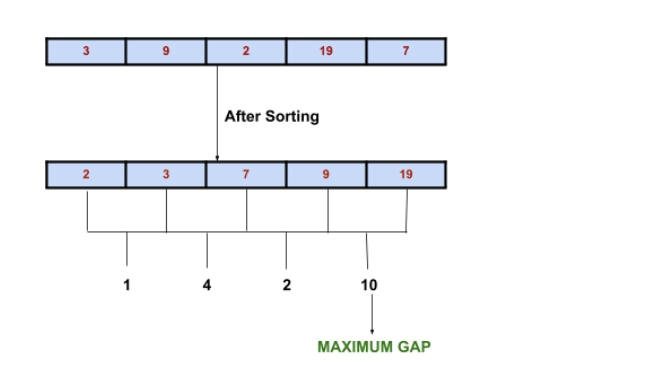New update is available. Click here to update.

# Maximum Gap

## Introduction

The task is to return the maximum difference between any two consecutive elements in the array after sorting it. Let us understand with an example.## Approach

The approach of the above algorithm is fairly simple. First, check if the length of the input array is at least 2. If not, return 0 since we can’t find the absolute difference if the size of the array is less than 2. After checking, we would sort the input array (preferably with merge sort or quick sort to keep time complexity as least as possible) and then find the absolute difference between all the consecutive elements in the array. We would initialise a temporary variable with a -1 value and then traverse the array finding the absolute differences between two consecutive elements. As we move, we substitute the value in a temporary variable if we come across a bigger absolute difference. We would then return the value after we traverse the array.

## Algorithm

1. Check the length of the input array.
2. If the length of the input array is <2, return 0. If not, follow the next steps.
3. Sort the input array.
4. Initialise a temporary variable with a -1 value.
5. Start traversing the array finding absolute differences between 2 consecutive elements. Store the value if it’s bigger than the value in the temporary variable.
6. After the traversal is complete, return the final value of the temporary value.

## Complexity Analysis

Time Complexity: If we exclude the time taken to sort the array (Assuming the input array is already in sorted order) then we would just have to traverse the array once. Hence time complexity of the algorithm is of the order O(N).

Space Complexity: The operations are all inplace, That is, there is no need for any auxiliary space. Hence the space complexity is of the order O(1).

Check out this array problem - Merge 2 Sorted Arrays

## FAQs

1. What are the time complexities of various sorting algorithms?

2. Which sorting technique is used in this algorithm?
We have used the in-built sorting function of python in our code. The in-built sorting algorithm is Tim Sort. It is an in-place sorting algorithm. It’s derived from merge sort and insertion sort. Hence its best case time complexity is of the order O(N) and the average case is of the order O(N log N).

3. For an already sorted array, which would be a better sorting technique - Merge Sort or Insertion Sort?
While Merge Sort has a better average and worst time complexity than insertion sort and is generally considered a better sorting technique, in this case, Insertion sort would prove to be more efficient. Since Insertion sort has the best case time complexity of the order O(N) while merge sort has O(N log N) in every scenario.

## Key Takeaways

It’s a basic algorithm to strengthen our core knowledge of different sorting techniques. Mastery of all the major sorting techniques is expected from a candidate aiming to join top product-based companies in the world. It’s essential to have an idea about where to use a certain sorting technique. Insertion sort, when applied on an already sorted array, would have a time complexity of the order O(N). While merge sort, which is considered a better sorting technique, would have a time complexity of the order O(N logN).

Happy Learning!